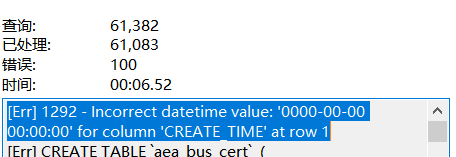C语言入门系列之9.预处理

# 一、无参宏定义

## 1.基本使用

``#define 标识符 字符串``

``````#include <stdio.h>

#define PI 3.1415926535

int main(){
double s;
int r;
scanf("%d", &r);
s = PI * r * r;
printf("s = %6.2lf", s);

return 0;
} ``````

``````Please input the radius:
12
s = 452.39``````

## 2.注意事项

（1）宏定义是用宏名来表示一个字符串，在宏展开时又以该字符串取代宏名，这只是一种简单的代换，字符串中可以包含任何字符，可以是常数，也可以是表达式，预处理程序对它不作任何检查。如有错误，只能在编译已被宏展开后的源程序时发现。

（2）宏定义不是说明或语句，在行末不必加分号，如加上分号则连分号也一起置换。

（3）宏定义必须写在函数之外，其作用域为宏定义命令起到源程序结束，如要终止其作用域可使用`#undef`命令。

（4）宏名在源程序中若用引号括起来，则预处理程序不对其作宏代换。

``````#include <stdio.h>

#define PI 3.1415926535

int main(){
void func(void);
double s;
int r;
scanf("%d", &r);
s = PI * r * r;
printf("s = %6.2lf\n", s);
func();

return 0;
}

void func(void){
printf("Now the PI = %g\n", PI);
printf("PI");
}``````

``````Please input the radius:
12
s = 452.39
Now the PI = 3.14159
PI``````

（5）宏定义允许嵌套，在宏定义的字符串中可以使用已经定义的宏名。 在宏展开时由预处理程序层层代换。

``````#include <stdio.h>

#define PI 3.1415926535
#define S PI*r*r

int main(){
void func(void);
double s;
int r;
scanf("%d", &r);
s = S;
printf("s = %6.2lf\n", s);

return 0;
}``````

（6）习惯上宏名用大写字母表示，以便于与变量区别。 但也允许用小写字母。

（7）可用宏定义表示数据类型，使书写方便。 例如`#define INTEGER int`

``````#include <stdio.h>

#define PIN1 char*
typedef char* PIN2;

int main(){
PIN1 x, y;
PIN2 a, b;
printf("By #define: %d %d\n", sizeof(x), sizeof(y));
printf("By typedef: %d %d\n", sizeof(a), sizeof(b));

return 0;
}``````

``````By #define: 8 1
By typedef: 8 8
``````

（8）对输出格式作宏定义，可以减少书写麻烦。

``````#include <stdio.h>

#define P printf
#define D "%d\n"
#define F "%f\n"

int main(){
int a = 3, c = 4, e = 5;
float b = 1.2, d = 2.3, f = 3.4;
P(D F, a, b);
P(D F, c, d);
P(D F, e, f);

return 0;
}``````

``````3
1.200000
4
2.300000
5
3.400000
``````

# 二、带参宏定义

## 1.基本概念

C语言允许宏带有参数。 在宏定义中的参数称为形式参数，在宏调用中的参数称为实际参数。

``#define  宏名(形参表)  字符串``

``宏名(实参表);``

``````#define M(y) y*y+3*y      /*宏定义*/
……
k=M(5);                   /*宏调用*/
……``````

``````#include <stdio.h>

#define MAX(a, b) (a > b) ? a:b

int main(){
int x, y, max;
printf("Input 2 numbers:\n");
scanf("%d %d", &x, &y);
max = MAX(x, y);
printf("Max = %d\n", max);

return 0;
}``````

``````Input 2 numbers:
12 20
Max = 20
``````

## 2.注意事项

（1）带参宏定义中，宏名和形参表之间不能有空格出现。 例如把`#define MAX(a,b) (a>b)?a:b`写为`#define MAX (a,b) (a>b)?a:b`会报错。

（2）在带参宏定义中，形式参数不分配内存单元，因此不必作类型定义； 而宏调用中的实参有具体的值，要用它们去代换形参，因此必须作类型说明。 这与函数中的情况是不同的： 在函数中，形参和实参是两个不同的量，各有自己的作用域，调用时要把实参值赋予形参，进行值传递； 而在带参宏中，只是符号代换，不存在值传递的问题。

（3）在宏定义中的形参是标识符，而宏调用中的实参可以是表达式。

``````#include <stdio.h>

#define SAY(y) y+5

int main(){
int i = 0;
char say[] = {73, 52, 108, 111, 118, 101, 32, 102, 105, 115, 104, 79, 46, 99, 91, 109};
while(say[i]){
say[i] = SAY(say[i] * 0.8);
i++;
}
printf("%s\n", say);

return 0;
}``````

``````?.[]cUVYaXD)TM\e
``````

（4）在宏定义中，字符串内的形参通常要用括号括起来以避免出错。

``````#include <stdio.h>

#define SQ1(y) (y)*(y)
#define SQ2(y) y*y

int main(){
int a, sq1, sq2;
printf("Input a number:\n");
scanf("%d", &a);
sq1 = SQ1(a+1);
sq2 = SQ2(a+1);
printf("SQ1 = %d\nSQ2 = %d\n", sq1, sq2);

return 0;
}``````

``````Input a number:
3
SQ1 = 16
SQ2 = 7
``````

``````#include <stdio.h>

#define SQ1(y) (y)*(y)
#define SQ2(y) ((y)*(y))

int main(){
int a, sq1, sq2;
printf("Input a number:\n");
scanf("%d", &a);
sq1 = 32 / SQ1(a+1);
sq2 = 32 / SQ2(a+1);
printf("SQ1 = %d\nSQ2 = %d\n", sq1, sq2);

return 0;
}``````

``````Input a number:
3
SQ1 = 32
SQ2 = 2
``````

（5）带参的宏和带参函数很相似，但有本质上的不同，除上面已谈到的各点外，把同一表达式用函数处理与用宏处理两者的结果有可能是不同的。

``````#include <stdio.h>

int main(){
int i = 1;
int SQ(int y);
while(i <= 5){
printf("%d\n", SQ(i++));
}

return 0;
}

int SQ(int y){
return ((y) * (y));
}``````

``````1
4
9
16
25
``````

``````#include <stdio.h>

#define SQ(y) ((y)*(y))

int main(){
int i = 1;
while(i <= 5){
printf("%d\n", SQ(i++));
}

return 0;
}``````

``````2
12
30
``````

（6）宏定义也可用来定义多个语句，在宏调用时，把这些语句又代换到源程序内。

``````#include <stdio.h>
#include <string.h>

#define STR(s1, s2, s3, sum) strcat(strcat(strcat(sum, s1), s2), s3)

int main(){
char str1[] = "I ", str2[] = "love ", str3[] = "C!", str = "";
STR(str1, str2, str3, str);
printf("str1 = %s\nstr2 = %s\nstr3 = %s\nstr  = %s\n", str1, str2, str3, str);

return 0;
}``````

``````str1 = I
str2 = love
str3 = C!
str  = I love C!
``````

``````#include <stdio.h>
#include <string.h>

#define STR(s1, s2, s3, sum) strcat(strcat(strcat(sum, s1), s2), s3)

int main(){
char str1[] = "I ", str2[] = "love ", str3[] = "C!", str = "";
STR(str1, str2, str3, str);
printf("str1 = %s\nstr2 = %s\nstr3 = %s\nstr  = %s\n", str1, str2, str3, str);

str = 0;
STR(str3, str1, str2, str);
printf("\nstr1 = %s\nstr2 = %s\nstr3 = %s\nstr  = %s\n", str1, str2, str3, str);

return 0;
}``````

``````str1 = I
str2 = love
str3 = C!
str  = I love C!

str1 = I
str2 = love
str3 = C!
str  = C!I love
``````

# 三、文件包含

1. 一个include命令只能指定一个被包含文件，若有多个文件要包含，则需用多个include命令。

2. 文件包含允许嵌套，即在一个被包含的文件中又可以包含另一个文件。

3. 包含命令中的文件名可以用双引号括起来，也可以用尖括号括起来。例如以下写法都是允许的：

``````#include "stdio.h"
#include <math.h>``````

但是这两种形式是有区别的： 使用尖括号表示在包含文件目录中去查找(包含目录是由用户在配置环境时设置的)，而不在源文件目录去查找； 使用双引号则表示首先在当前的源文件目录中查找，若未找到才到包含目录中去查找。

# 四、条件编译

1. 第一种形式：

``````#ifdef  标识符
程序段1
#else
程序段2
#endif``````

它的功能是，如果标识符已被`#define`命令定义过则对程序段1进行编译；否则对程序段2进行编译。 如果没有程序段2(为空)，本格式中的`#else`可以没有，即可以写为：

``````#ifdef  标识符
程序段
#endif``````
2. 第二种形式：

``````#ifndef  标识符
程序段1
#else
程序段2
#endif``````

``````#include <stdio.h>

#define CORRECT "corley.com"

int main(){
char str;
int cmp(char *str1, char *str2);
printf("Input the website you like best:\n");
scanf("%s", str);

#ifndef CORRECT
#define CORRECT "corley.com"
#endif

if(cmp(str, CORRECT) == 0){
printf("You are so cute!\n");
}
else{
}

return 0;
}

int cmp(char *str1, char *str2){
int i = 0, j = 0;
while(str1[i]){
while(str2[j] == str1[i]){
i++;
j++;
if(!str2[j]){
return 0;
}
}
j = 0;
i++;
}
return -1;
}``````

``````Input the website you like best:
corley.com
You are so cute!
``````
1. 第三种方式：
``````#if  常量表达式
程序段1
#else
程序段2
#endif``````

``````#include <stdio.h>

#define ROUND 1
#define PI 3.14159

int main(){
int r;
double s;
scanf("%d", &r);

#if ROUND
s = r * r * PI;
printf("Area of circle is: %6.2f\n", s);
#else
s = r * r;
printf("Area of square is: %6.2f\n", s);
#endif

return 0;
}``````

``````Input the radius:
12
Area of circle is: 452.39
``````

# 五、预处理小结

1. 预处理功能是C语言特有的功能，它是在对源程序正式编译前由预处理程序完成的，开发者在程序中用预处理命令来调用这些功能。

2. 宏定义是用一个标识符来表示一个字符串，这个字符串可以是常量、变量或表达式。 在宏调用中将用该字符串代换宏名。

3. 宏定义可以带有参数，宏调用时是以实参代换形参，而不是值传送

4. 为了避免宏代换时发生错误，宏定义中的字符串应加括号，字符串中出现的形式参数两边也应加括号。

5. 文件包含是预处理的一个重要功能，它可用来把多个源文件连接成一个源文件进行编译，结果将生成一个目标文件。

6. 条件编译允许只编译源程序中满足条件的程序段，使生成的目标程序较短，从而减少了内存的开销并提高了程序的效率。

7. 使用预处理功能便于程序的修改、阅读、移植和调试，也便于实现模块化程序设计。blmius
1年前
MySQL:[Err] 1292 - Incorrect datetime value: ‘0000-00-00 00:00:00‘ for column ‘CREATE_TIME‘ at row 1Jacquelyn38
2年前
2020年前端实用代码段，为你的工作保驾护航helloworld_34035044
11个月前

​在我们用皕杰报表工具设计填报报表时，如何在新增行里自动增加id呢？能新增整数排序id吗？目前可以在新增行里自动增加id，但只能用uuid函数增加UUID编码，不能新增整数排序id。uuid函数说明：获取一个UUID，可以在填报表中用来创建数据ID语法：uuid()或uuid(sep)参数说明：sep布尔值，生成的uuid中是否包含分隔符''，缺省为Wesley13
1年前
FLV文件格式
1.        FLV文件对齐方式FLV文件以大端对齐方式存放多字节整型。如存放数字无符号16位的数字300（0x012C），那么在FLV文件中存放的顺序是：|0x01|0x2C|。如果是无符号32位数字300（0x0000012C），那么在FLV文件中的存放顺序是：|0x00|0x00|0x00|0x01|0x2C。2.Stella981
1年前
KVM调整cpu和内存Easter79
1年前Wesley13
1年前
mysql设置时区
mysql设置时区mysql\_query("SETtime\_zone'8:00'")ordie('时区设置失败，请联系管理员！');中国在东8区所以加8方法二：selectcount(user\_id)asdevice,CONVERT\_TZ(FROM\_UNIXTIME(reg\_time),'08:00','0Stella981
1年前Stella981
1年前
Nginx反向代理upstream模块介绍
!(https://oscimg.oschina.net/oscnet/1e67c46e359a4d6c8f36b590a372961f.gif)!(https://oscimg.oschina.net/oscnet/819eda5e7de54c23b54b04cfc00d3206.jpg)1.Nginx反Wesley13
1年前
MySQL部分从库上面因为大量的临时表tmp_table造成慢查询CuterCorley
Lv1

108

1

6